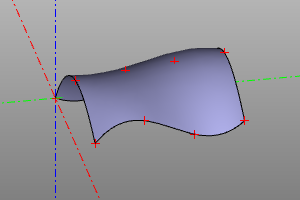# Flat primitives.

This section introduces flat primitives. They are usually used in conjunction with 3D operations to construct bodies with complex geometry.

## Rectangle

The flat primitive is a rectangle. Set by two sides. It is permissible not to indicate the second side, which will correspond to the construction of the square. Setting the center option aligns the geometric center of the body with the origin. When the wire option is set, a rectangle loop will be generated instead of a filled face.

Signature:

``````rectangle(x, y, center=True/False, wire=True/False)
rectangle(a, center=True/False, wire=True/False)
square(a, center=True/False, wire=True/False) #alternate
``````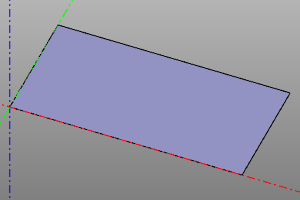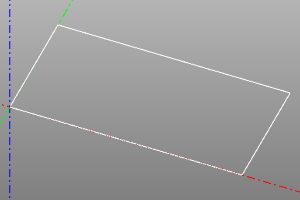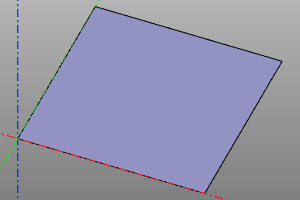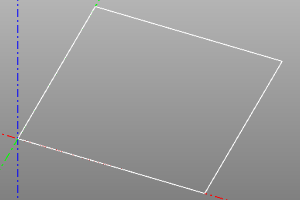## Circle / Circle

The circle is given by the radius r. Setting the optional angle option allows you to generate a sector of a circle / arc of a circle. When the wire option is set, a wireframe circle will be generated instead of a filled circle face.

Signature:

``````circle(r=radius, wire=True/False)
``````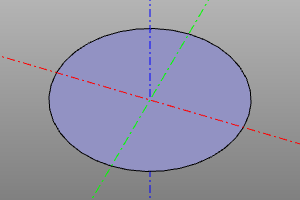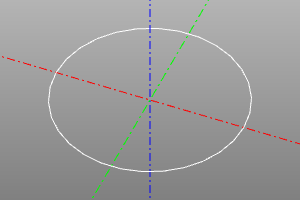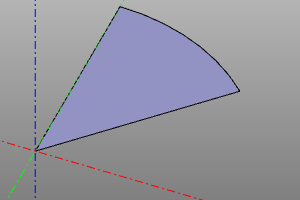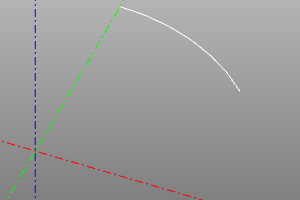## Ellipse

The flat primitive is an ellipse. It is specified by two radii, and r1 must be greater than r2. You can also draw a wedge by specifying an angle or a pair of angles as the optional angle parameter. When the wire option is set, a wireframe will be generated instead of a filled face.

Signature:

``````ellipse(r1=major, r2=minor, wire=True/False)
ellipse(r1=major, r2=minor, angle=angle, wire=True/False)
ellipse(r1=major, r2=minor, angle=(start, stop), wire=True/False)
``````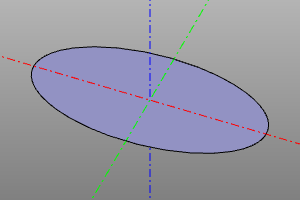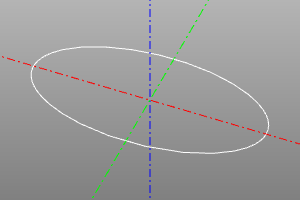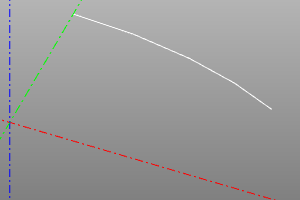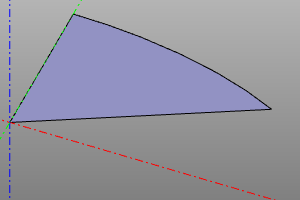## Polygon

A flat primitive is a polygon. Constructed by vertex points. When the wire option is set, a wireframe will be generated instead of a filled face (which is similar to a closed polysegment.). pnts is an array of vertex points.

Signature:

``````polygon(pnts=pnts, wire=True/False)
``````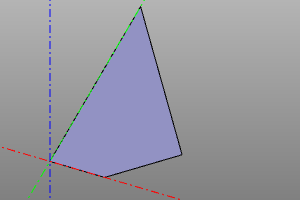## Regular polygon

A flat primitive is a regular polygon. The radius and the number of vertices are set. When the wire option is set, a wireframe will be generated instead of a filled face.

Signature:

``````ngon(r=radius, n=vertexCount, wire=True/False)
``````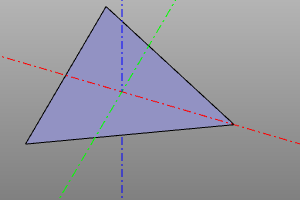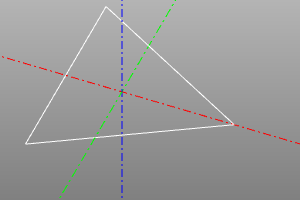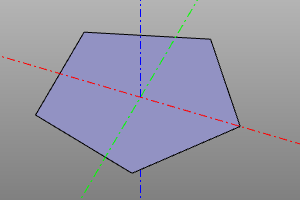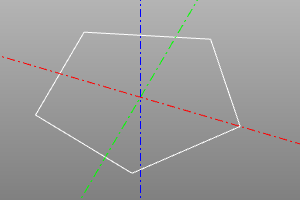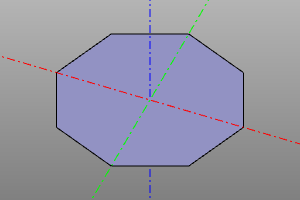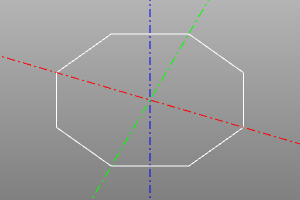## Text shape

The flat primitive is text. Creates a face based on string `text` and name of font `fontname` with font size `size`. The font is selected from those registered in the system. To register additional fonts use `register_font` command. The `composite_curve` option reduce the number of edges in the resulting shape by increasing their complexity.

Signature:

``````textshape(text, fontname, size, composite_curve=False)
``````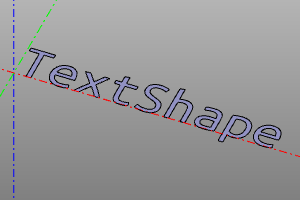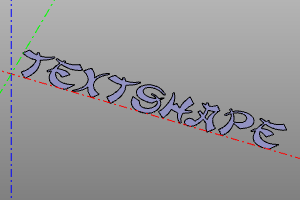## Infinite Plane

An infinite plane is a special geometric object that can be used in some operations on other objects. An infinite plane cannot be displayed directly.

Signature:

``````infplane()
``````

Пример (Построение конических сечений):

``````cone(r1=5, r2=0, h=10, center=True) ^ infplane()
cone(r1=5, r2=0, h=10, center=True).rotX(deg(45)) ^ infplane()
cone(r1=5, r2=0, h=10, center=True) ^ infplane().rotX(deg(45))
cone(r1=5, r2=0, h=10, center=True) ^ infplane().rotX(deg(90)).right(3)
``````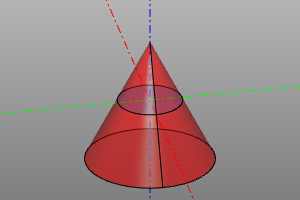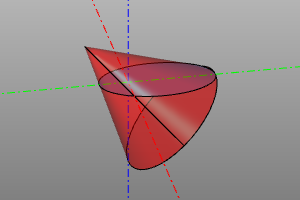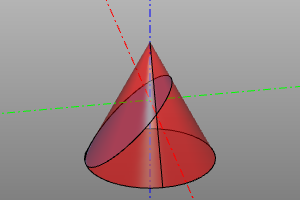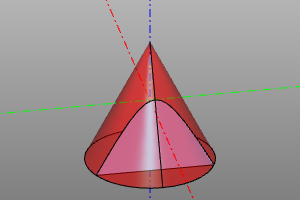## Filling the outline

This operation is applied to the flat closed line wire and turns it into a face.

Signature:

``````fill(wire)
wire.fill() #alternate
``````

Example:

``````wire = sew([
segment((0,0,0), (0,10,0)),
circle_arc((0,10,0),(10,15,0),(20,10,0)),
segment((20,0,0), (20,10,0)),
segment((20,0,0), (0,0,0))
])
fill(wire)
``````
До После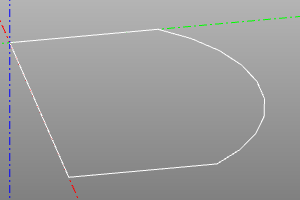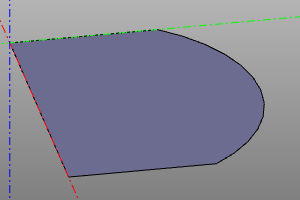## Interpolate a surface over an array of points

Builds a bspline surface by interpolating a 2D array of points. The array is specified by a two-dimensional list. degmin and degmax define the minimum and maximum degrees of the interpolation polynomial, respectively.

Signature:

``````interpolate2(pnts, degmin=3, degmax=7)
``````

Example:

``````POINTS = points2([
[(0,0,0), (10,0,7), (20,0,5)],
[(0,5,0), (10,5,7.5), (20,5,7)],
[(0,10,2), (10,10,8), (20,10,5)],
[(0,15,1.3), (10,15,8.5), (20,15,6)],
])

m = interpolate2(POINTS)
disp(m)
disp(POINTS, color=color.red)
``````